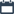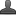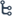## Tuesday, October 27, 2020

•October 27, 2020
••, ,
•No comments

## Important questions for 10th class Maths chapter-5 Quadratic equations,Mathematics questionnaire-x class

Hi friends and my dear students! In this post, I have covered Important questions for 10th class maths chapter-5 Quadratic-equations, Chapter wise previous year Questions. After Reading  Please do share it with your friends. Learn maths for All classes here

PRIORITY-I

1 MARK QUESTIONS:

1. Raju and Rajendar together have 45 marbles. Both of them lost 5 marbles each and the product of the number of marbles now they have is 124. Represent the situation in the form of quadratic equation to find out how many marbles they have previously?

2. The sum of the reciprocals of Rehman’s ages (in years) 3 year ago and 5 years from now is 1/3 find his present age.

3. Verify the following equations are quadratic or not? Explain?

i.(x+1/x )2 =3.(x+1/x)+4;

ii. (2x+1)(3x+2)=6 (x-1)(x-2);

iii. x2+2 x+3=0

4. Determine the nature of roots ofi) x2+x+1=0,ii. 2x2+x-1=0 iii. 4x2-4x+1=0

5. The hypotenuse of a right triangle is 25cm. we know that the difference in the lengths of the other two sides is 5cm. Represent the situation in the form of quadratic equation to find out the lengths of two sides?

6. The product of two consecutive positive integers is 306. Represent the situation in the form of quadratic equation to find the integers?

7. Verify that 1 and 3/2 are roots of 2x2-5x+3=0?

8. Find the roots of 2x2-5x+3=0 by factorization method?

9. Find the discriminant of 2x2-4x+3=0and hence find the nature of the roots?

10. Find the value of k for 2x2-kx+3=0,so that it has two equal roots ?

Also Check

## Important questions for 10th class maths chapter-1 Real numbersImportant questions for 10th class maths chapter-4 LINEAR EQUATIONSImportant questions for 10th class maths chapter-3 polynomial

2 MARKS QUESTIONS

11. Find two numbers whose sum is 27 and the product is 182?

12. The root of the following equations has real and equal roots, find the value k.

i. x2-2x(1+3k)+7(3+2k)=0

ii.(k+1)x2-2(k-1)x+1=0

13. Solve 2/x2-5/x +2=0 by factorization method.

14. Find the quadratic equation whose roots are 2+3 and 2-3

15. Check (x-2) is a factor of x3-4x2-x+1=0

16. Explain the benefits of evaluating the discriminant of a quadratic equation before attempting to solve it. What does it value signifies?

17. Find two consecutive positive integers, sum of whose square is 613?

18. Find the roots of i) = 1/x +4,1/x-7 =11/30x≠-4,7ii) 1/x-1/x-2=3  ≠0,2

19. find the roots of the equation i. 5x2-6x-2=0 by the method of completing the square ii.x2+7x-6=0, iii. x2-10x+9=0

20. the difference of squares of two numbers is 180 the square of the smaller number is 8 times the larger number find the two numbers

21. Sum of the area of two squares is 468m2 if the difference of their perimeters is 24m then find the sides of the two squares.

22. Write any two uses of quadratic equations?

23. Find roots of √2x2+7x+5√2=0

24. Find two consecutive odd positive integers, sum of whose square is 290?

25. Find the roots√2x2-2√2x+1=0 if they exist, using quadratic formula?

26. Find the roots of x+1/x=3

27. Find the discriminant of3x2-2x+1/3=0 and find the nature of its roots find them if they are real?

4 MARKS QUESTIONS:

1. Find the dimensions of the rectangle whose perimeter is 28m, and whose area is 40m2

2. The base of a triangle is 4cm longer than its altitude. If the area of triangle is 48cm2, then find its base and altitude?

3. It is possible to design a rectangular park of perimeter 80m and area 400m2? If so find its length and breadth

4. If a polygon of ‘n’ sides has 1/2n(n-3) diagonals. How many sides will a polygon having 65 diagonals? Is there a polygon with 50 diagonals?.

5. Two water taps together can fill a tank in 9 3/8hours. The tap of larger diameter takes 10 hours less than the smaller one to fill the tank separately find the time in which watch tap can separately fill the tank.

6. If The diagonal of a rectangular field is 60meters more than the shorter side. If the longer side is 30 metre more than the shorter side, find the sides of the field.

7. A ball is thrown vertically upward from the top of a building 96m tall with an initial velocity 80m/sec the distance ‘s’ of the ball from the ground after t seconds is S=96+80t-4.9t2 after how many seconds does the ball strike the ground.

8. A motor boat whose speed is 18km/h in still water. It takes 1 hour more to go 24km upstream than to return downstream to the same spot. Find the speed of the stream?

9. The altitude of a right triangle is 7m less than its base. If the hypotenuse is 13cm. find the other two sides?

10. A motor boat heads upstream a distance of 24km on a river whose current is running at 3 km per hour. The trip up and back takes 6 hours. Assuming that the motor boat maintained a constant speed, what was its speed?

11. Is it possible to design a rectangular mango grove whose length is twice its breadth, and the area is 800m2? If so, find its length and breadth?

## IITJEE 7th class Introduction to Algebra Notes

PRIORITY – II:

1 MARK QUESTIONS:

1. If the root of the equation x2+7x+k=0 is -1 then find the value of ‘k’ and other root?

2. The product of two consecutive odd integers is 63 represent this in the form of a quadratic equation

3. Give two examples for a quadratic equation which has no real roots

4. Find the roots of ? x√x =√xx

5. Draw the rough sketch of the graph of quadratic equation touching x-axis at one point?

6. If b2-4ac≥0, then write the roots of quadratic equation ax2+bx+c=0?

7. Find sum and product of the roots of the equation x2-4x+3=0

8. Why do we take ‘a’ common while making a quadratic equation ax2+bx+c=0 to complete a square

9. Find ‘x’ if i. 3+32x=4x3x ii. ax(x-1)=1/a-6

10. Find the value of ‘k’ for which the equation x2-4x+k=0 has distinct real roots.

2 MARKS QUESTIONS:

1. Find the roots of the equation x+1/x+41/4,x≠0?

2. Divide 51 into two parts such that their product is 378?

3. Find ‘K’ so that (k-12)x2+2(k-12)x+2=0 has equal roots.

4. Sum of two numbers is 15, if sum of their reciprocal is 3/10 find the numbers

5. Solve the equation a2x2+(a2-b2)x-b2=0

6. If x=2 and x=3 are roots of the equation 3x2-2k+2m=0 find the value of k and m.

7. Krishna told “every quadratic equation will have real roots” Raghav told 7x=9x2 is a quadratic equation who is correct? Justify your answer.

8. Find the value of ‘k’ if x2+x-k=2 and x2- 10x+(2k-3)=0 have 3 has a common root.

9. Find the quadratic equation whose roots are 2+3i and 2-3i

10. Find the value of k for the quadratic equation 9x2-kx+4=0 so that this has two equal roots.

11. If the roots of the quadratic equation kx(x-2)+6=0 are equal then find the value of ‘k’

12. If one root of the quadratic equation 2x2+kx-6=0 is 2, find the value of k also find the other root

13. Solve the quadratic equation x2-2ax+a2- b2=0 by factorization method.

14. Determine whether the quadratic equation 3x2+2√5 -5=0 have real roots and if so, find the roots

15. Find the roots of 9x2+7x-2=0 by using quadratic formula.

16. What are the methods to solve a quadratic equation?